# ESP32 - Potentiometer

This tutorial instructs you how to use ESP32 with the potentiometer. In detail, we will learn:

• How the potentiometer works
• How to interface ESP32 with a potentiometer
• How to program ESP32 to read the value from a potentiometer
• How to convert the value read from a potentiometer to the useful value

## Hardware Used In This Tutorial

Or you can buy the following sensor kit:

Disclosure: some of these links are affiliate links. We may earn a commission on your purchase at no extra cost to you. We appreciate it.

## Introduction to Potentiometer

The potentiometer (also known as rotary angle sensor) is used to change the settings (e.g. the speaker's volume, room's temperature, lamp's brightness...)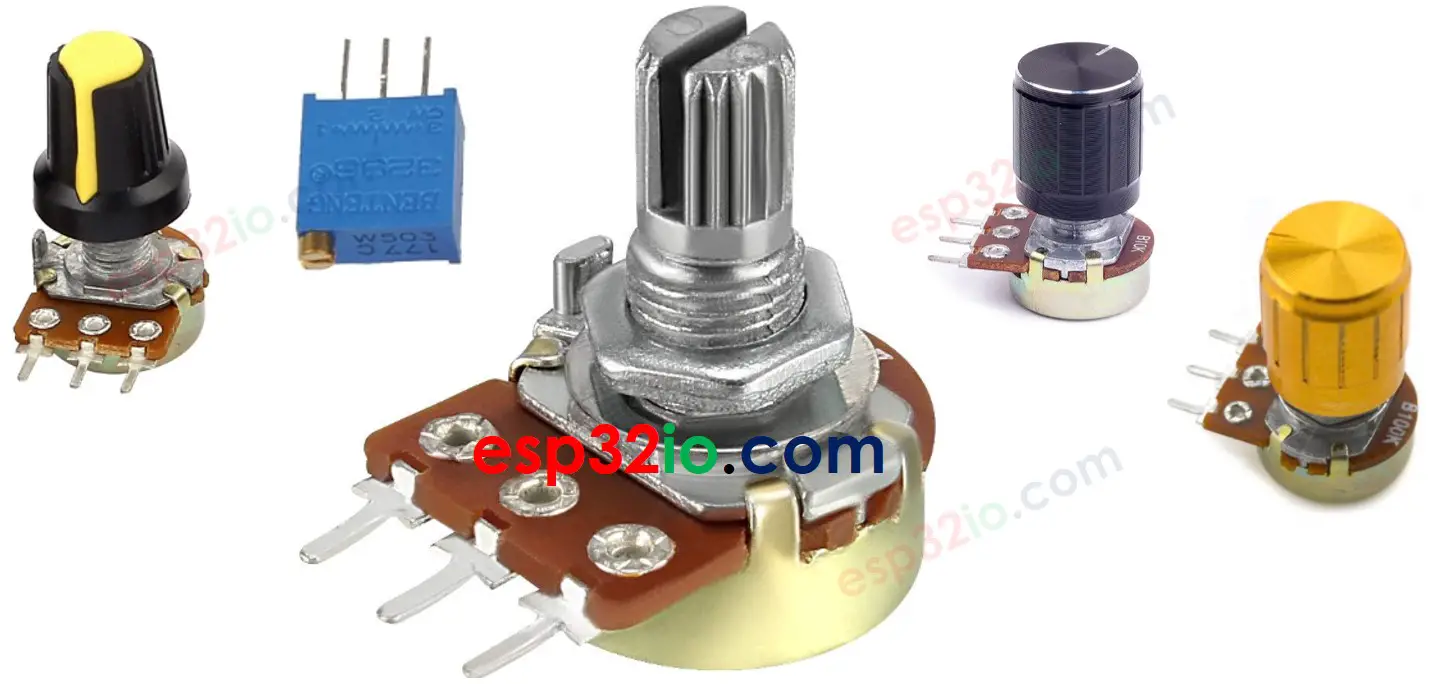### Potentiometer Pinout

A potentiometer usually has 3 pins:

• VCC pin: connect this pin to VCC (5V or 3.3v)
• GND pin: connect this pin to GND (0V)
• Output pin: outputs the voltage to ESP32's input pin.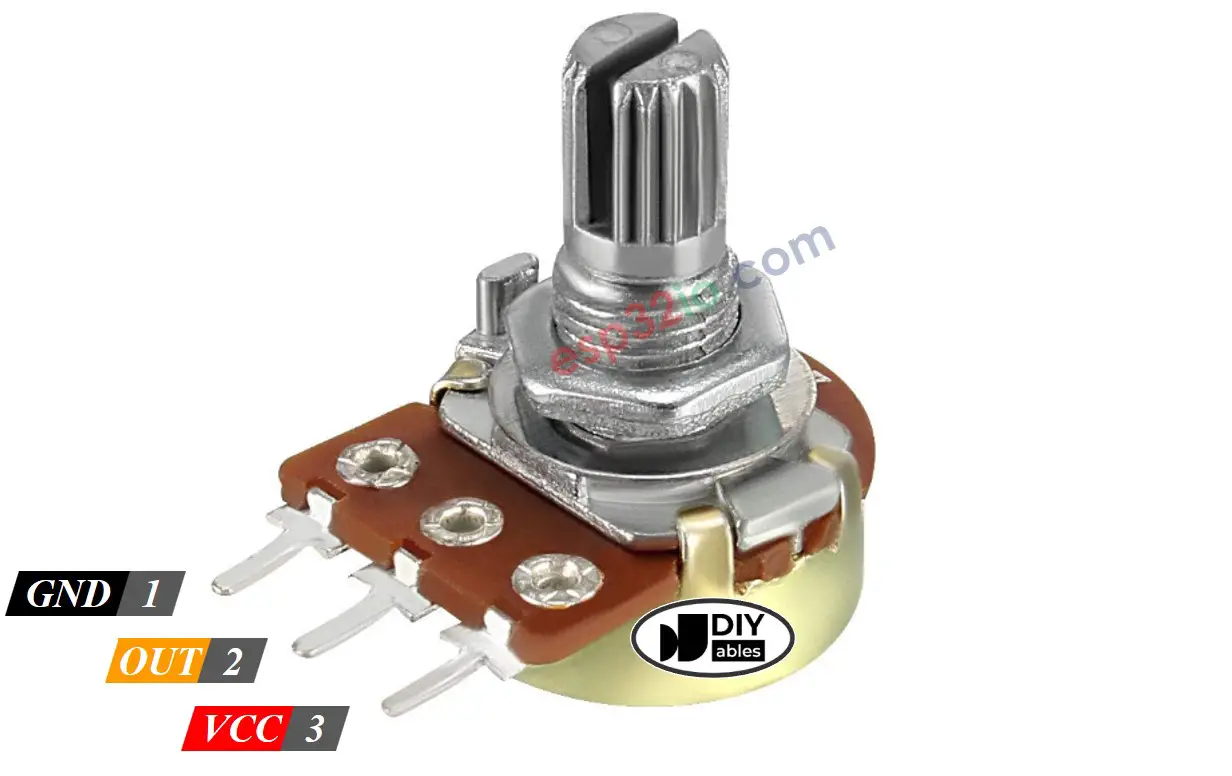※ NOTE THAT:

The GND pin and VCC pin are interchangeable

### How Potentiometer Works

The potentiometer's shaft can be rotated from 0° (closest to GND pin) to an angle (closest to VCC pin), called ANGLE_MAX.

The voltage in the output pin is in direct proportion to the angle position of the shaft, varing from 0 to VCC. The below table shows the relation between the angle and the voltage on the output pin:

Angle Voltage
0v
ANGLE_MAX°VCC
angle°angle° × VCC / ANGLE_MAX°

※ NOTE THAT:

The ANGLE_MAX value is depended on manufacturers.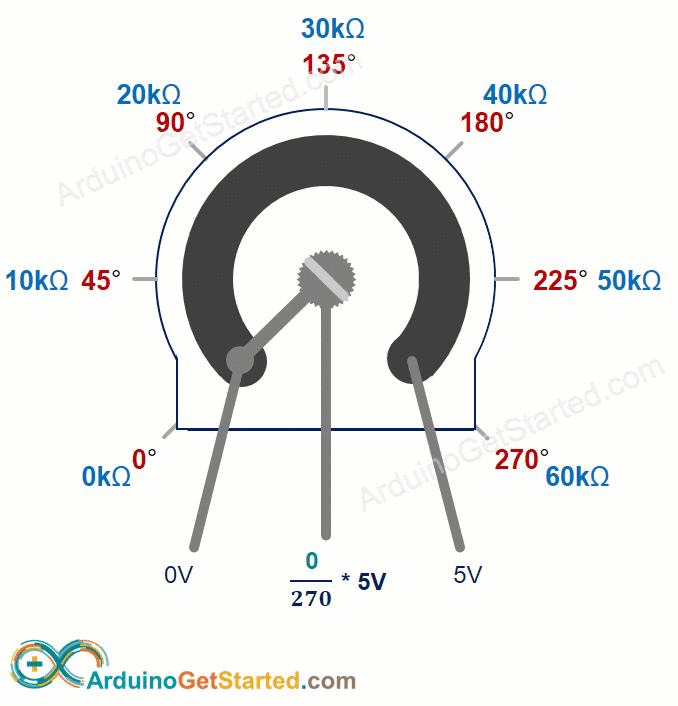### ESP32 - Rotary Potentiometer

The ESP32's analog input pin converts the voltage (between 0v and 3.3V) into integer values (between 0 and 4095), called ADC value or analog value.

We can connect the potentiometer's output pin to an ESP32's analog input pin, and then read the analog value from the pin.

The analog value from input pin can be rescaled into another value. Let's see the use cases:

• Rescale the analog value to the potentiometer's angle.
• Rescale the analog value to the potentiometer's voltage.
• Rescale the analog value to the the setting value (e.g. the speaker's volume, room's temperature, lamp's brightness...)

#### Rescale Range

FROM TO
Anglerotated by userANGLE_MAX°
Voltagefrom potentiometer's pin 0V3.3V
Setting valueconverted by ESP32 VALUE_MINVALUE_MAX

## Wiring Diagram between Potentiometer and ESP32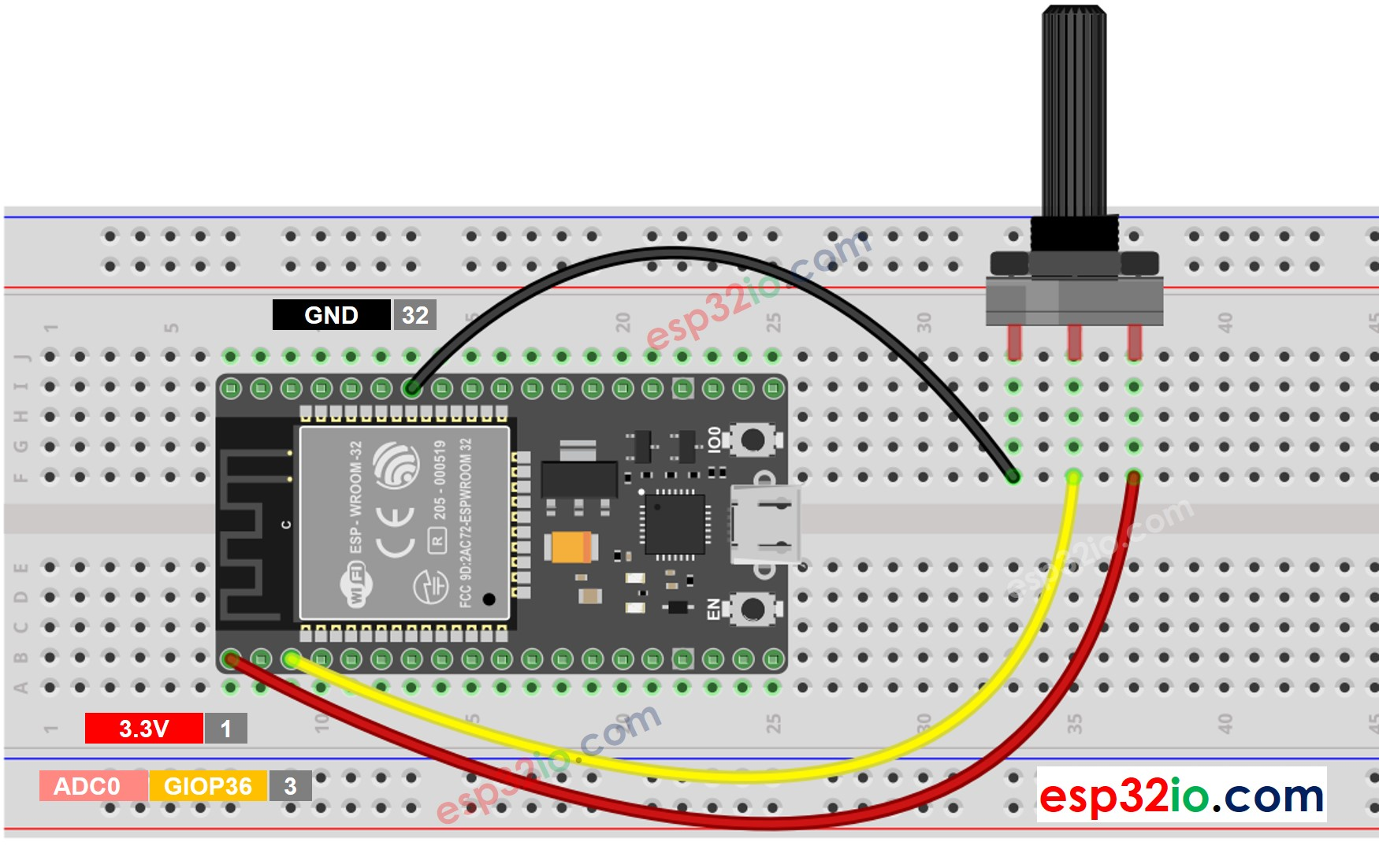This image is created using Fritzing. Click to enlarge image

If you're unfamiliar with how to supply power to the ESP32 and other components, you can find guidance in the following tutorial: How to Power ESP32.

## How To Program Potentiometer

• Read the value from an input pin, which connected to the output pin of the potentiometer by using analogRead() function.
• Rescale to the potentiometer's angle by using map() function.
angle = map(analogValue, 0, 4095, 0, ANGLE_MAX);
• Rescale to the potentiometer's voltage:
voltage = map(analogValue, 0, 4095, 0, 3.3);
• Rescale to the controllable value (e.g volume of stereo, brightness, speed of DC motor... )
value = map(analogValue, 0, 4095, VALUE_MIN, VALUE_MAX);
• For example, rescaling to the brightness of LED. As mentioned in this tutorial, the brightness of LED can be controlled by using PWM value from 0 (always OFF) to 255 (always ON). Therefore, we can map the analog value to the brightness of LED (from OFF to the brightest) as follows:
brightness = map(analogValue, 0, 4095, 0, 255);

If you want to dim LED from the nightlight to the brightest,

nightlight = 100; // depending on your desired brightness brightness = map(analogValue, 0, 4095, nightlight, 255);

※ NOTE THAT:

The map() function can only be used to rescale the analog value to the int or long type value. If the controllable value is float type, you need to use the floatMap() function instead of the map() function.

floatMap() function:

float floatMap(float x, float in_min, float in_max, float out_min, float out_max) { return (x - in_min) * (out_max - out_min) / (in_max - in_min) + out_min; }

## ESP32 Code

/* * This ESP32 code is created by esp32io.com * * This ESP32 code is released in the public domain * * For more detail (instruction and wiring diagram), visit https://esp32io.com/tutorials/esp32-potentiometer */ float floatMap(float x, float in_min, float in_max, float out_min, float out_max) { return (x - in_min) * (out_max - out_min) / (in_max - in_min) + out_min; } // the setup routine runs once when you press reset: void setup() { // initialize serial communication at 9600 bits per second: Serial.begin(9600); } // the loop routine runs over and over again forever: void loop() { // read the input on analog pin GPIO36: int analogValue = analogRead(36); // Rescale to potentiometer's voltage (from 0V to 3.3V): float voltage = floatMap(analogValue, 0, 4095, 0, 3.3); // print out the value you read: Serial.print("Analog: "); Serial.print(analogValue); Serial.print(", Voltage: "); Serial.println(voltage); delay(1000); }

### Quick Instructions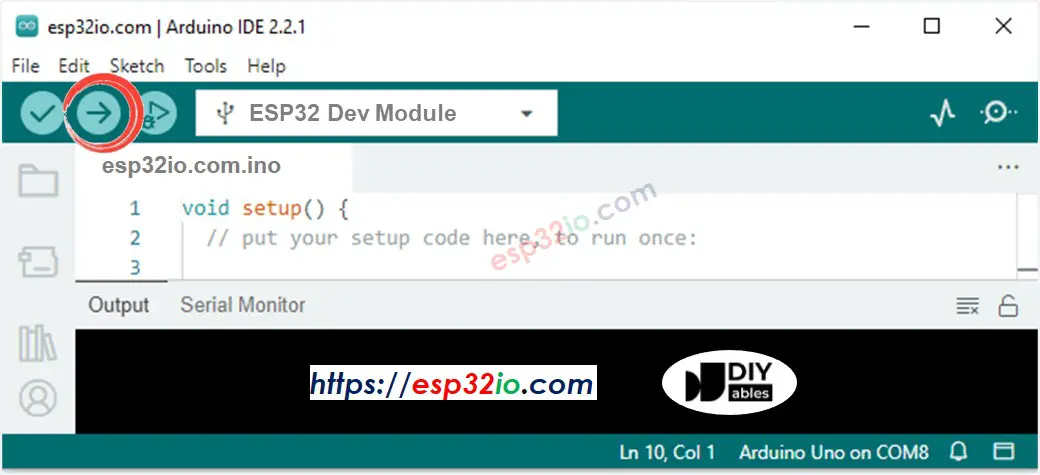• Open Serial Monitor on Arduino IDE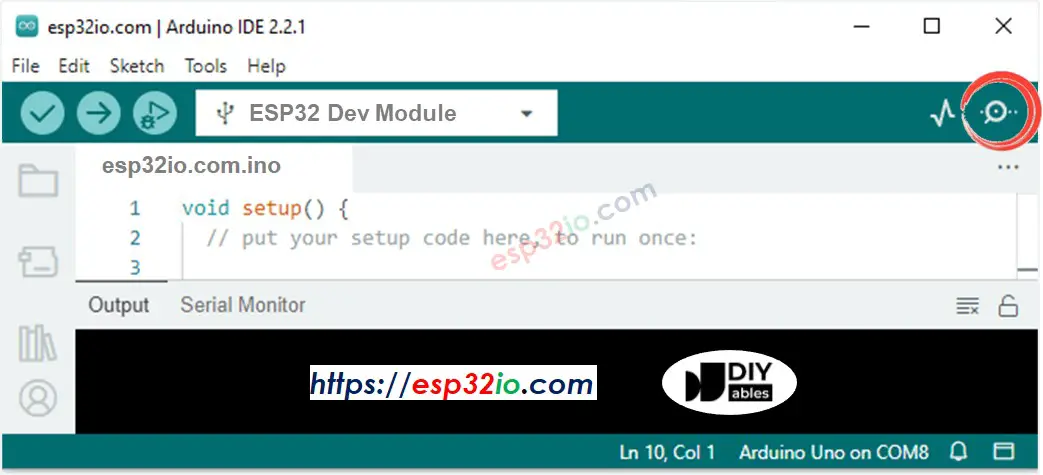• Rotate the potentiometer
• See the result on Serial Monitor. It looks like the below:
COM6
Analog: 0, Voltage: 0.00 Analog: 0, Voltage: 0.00 Analog: 126, Voltage: 0.62 Analog: 281, Voltage: 1.37 Analog: 517, Voltage: 2.53 Analog: 754, Voltage: 3.69 Analog: 906, Voltage: 4.43 Analog: 4095, Voltage: 3.30 Analog: 4095, Voltage: 3.30
Autoscroll Show timestamp
Clear output
9600 baud
Newline

## Video Tutorial

Making video is a time-consuming work. If the video tutorial is necessary for your learning, please let us know by subscribing to our YouTube channel , If the demand for video is high, we will make the video tutorial.

※ OUR MESSAGES